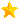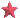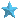### Magnitudes and distanceFor those who really want to understand the details, this primer describes the magnitude system and derives all of the equations relating magnitudes to distances.

Apparent magnitude
Absolute magnitude
Distance modulus
Interstellar reddening

#### Apparent magnitudeThe magnitude system began harmlessly enough. Scientists like to classify objects, and the Greek astronomer Hipparchus (160-127 B.C.) grouped the visible stars into six classes based on their apparent brightness. The brightest stars he called first-class stars, the next faintest he called second-class stars, and so on (Seeds 1997). The brightness classes are now known as apparent magnitudes, and are denoted by a lowercase m. The system is counterintuitive in that we naturally associate larger numbers with increasing brightness. The magnitude system uses the reverse philosophy -- a first magnitude star is brighter than a sixth magnitude star. Although the magnitude system can be awkward, it is so deeply ingrained in astronomical literature (and databases) that it would be difficult to abandon the system now.

There were no binoculars or telescopes in the time of Hipparchus. The original classification system was based on naked-eye observations, and was fairly simple. Under the best observing conditions, the average person can discern stars as faint as sixth magnitude. The light pollution in Chicagoland limits our view of the night sky at Northwestern. On a typical clear night in Evanston, you can see 3rd to 4th magnitude stars. On the very best nights, you might be able to see 5th magnitude stars. Although the loss of one or two magnitudes does not seem like much, consider that most of the stars in the sky are dimmer than 5th magnitude. The difference between a light polluted sky and dark sky is astonishing.

As instruments were developed which could measure light levels more accurately than the human eye, and telescopes revealed successively dimmer stars, the magnitude system was refined. A subscript may be added to the apparent magnitude to signify how the magnitude was obtained. The most common magnitude is the V magnitude, denoted mV, which is obtained instrumentally using an astronomical V filter. The V filter allows only light near the wavelength of 550 nanometers to pass through it (approximately 505-595 nm). V magnitudes are very close to those perceived by the human eye. (To what range of wavelengths is the human eye sensitive?) The following chart lists the apparent V magnitudes of a few common celestial objects.

Table 1: Some common apparent magnitudes (mV)

 Object mV Sun -26.8 Full Moon -12.5 Venus at brightest -4.4 Jupiter at brightest -2.7 Sirius -1.47 Vega 0.04 Betelgeuse 0.41 Polaris 1.99 Naked eye limit 6 Pluto 15.1 Hubble Space Telescope 31
Magnitudes from Seeds (1997), Kaufmann (1996), and Burnham (1978).

While you may perceive one star to be only a few times brighter than another, the intensity of the two stars may differ by orders of magnitude. (Light intensity is defined as the amount of light energy striking each square cm of surface per second.) The eye is a logarithmic detector. While the eye is perceiving linear steps in brightness, the light intensity is changing by multiplicative factors. This is fortunate; if the eye responded linearly instead of logarithmically to light intensity, you would be able to distinguish objects in bright sunlight, but would be nearly blind in the shade! If logarithms are a faint memory, you should peruse a refresher on logs and logarithmic scales before continuing.

Given that the eye is a logarithmic detector, and the magnitude system is based on the response of the human eye, it follows that the magnitude system is a logarithmic scale. In the original magnitude system, a difference of 5 magnitudes corresponded to a factor of roughly 100 in light intensity. The magnitude system was formalized to assume that a factor of 100 in intensity corresponds exactly to a difference of 5 magnitudes. Since a logarithmic scale is based on multiplicative factors, each magnitude corresponds to a factor of the 5th root of 100, or 2.512, in intensity. The magnitude scale is thus a logarithmic scale in base 1001/5 = 2.512. The following table illustrates the point.

Table 2: Magnitudes and intensity

 Magnitude difference Relative intensity 0 1 1 2.51 2 6.31 3 15.8 4 39.8 5 100 10 104 15 106

To reiterate, each magnitude corresponds to a factor of 2.512 in intensity, and each 5 magnitudes corresponds to a difference in 100 in intensity. A few examples will help clarify the point.

• Q: Star A has an apparent visual magnitude of 7, and its light intensity is 100 times dimmer than that of star B. What is the apparent visual magnitude of star B?

A: The intensities differ by a factor of 100, which means that the difference in magnitudes must be 5. Since A is dimmer than B, and brighter stars have lower magnitudes, the apparent visual magnitude of B must be 7 - 5 = 2. Star B is thus a second magnitude star.

• Q: Star A has a magnitude of 2.5, and star B has a magnitude of 4.5. What is the ratio of the intensities IA/IB, where IA is the intensity of star A, and IB is the intensity of star B?

A: The difference in magnitudes between the two stars is 4.5 - 2.5 = 2, so the intensities must differ by a factor of 2.512 squared, or 6.31 (see Table 2). Star A is brighter, because its magnitude is a smaller number. IA/IB should thus be larger than 1. It follows that IA/IB = 6.31.

Sometimes the numbers are not this simple, and we need general equations. How can we mathematically describe the relationship between intensity ratios and magnitude differences? While the magnitudes are increasing linearly, the intensity ratios are increasing logarithmically in base 2.512. Denoting magnitudes by m, intensities by I, and using subscripts A and B to denote stars A and B, we can express the relationship between intensity and magnitudes as follows:

IA / IB = (2.512)(mB - mA).

Convince yourself that this equation describes the numbers in Table 2. This form of the relationship is best when you know the relative magnitudes, and want to calculate the intensity ratio. We can manipulate the equation to put it in a more convenient form for the case when we know the intensities, but wish to find the relative magnitudes. Taking the log of both sides we get

log10(IA / IB) = log10 (2.512)(mB - mA).

Recalling from the general rules for logs that log10 MP = p log10 M, we can rewrite this as

log10(IA / IB) = (mB - mA) log10 2.512

or

log10(IA / IB) = 0.4 (mB - mA).

The result is most commonly expressed in the form

mB - mA = 2.5 log10 (IA / IB).

Let's do one more example of the magnitude system, this time using the equations.

• Q: The intensity of star B is a factor of 10 higher than that of star A. Star A has a magnitude of 2.4. What is the magnitude of star A?

A: First of all, think through the problem intuitively. Which star is brighter? Estimate by how many magnitudes the stars should differ. Should B have a higher or a lower magnitude?

Star B is brighter, so it should have a lower magnitude than A. If the intensities differ by a factor of 10, Table 2 shows that the difference in magnitudes should be somewhere between 2 and 3. Since mA = 2.4, the magnitude of B must therefore lie between 0.4 and -1.4 magnitudes. Now let's use the equations.

mB - mA = 2.5 log10 (IA / IB).

mB = mA + 2.5 log10 (IA / IB)

mB = 2.4 + [ 2.5 log10 (0.1) ]

mB = 2.4 + [ 2.5 (-1) ]

mB = -0.1

Our answer lies within the predicted range.

Whew!

#### Absolute magnitudeApparent magnitudes describe how bright stars appear to be. However, they tell us nothing about the intrinsic brightness of the stars. Why? The apparent brightness of a star depends on two factors: the intrinsic brightness of the star, and the distance to the star. The Sun is not particularly bright as stars go, but it appears spectacularly bright to us because we live so close to it.

If we want to compare the intrinsic brightness of stars using the magnitude system, we have to level the playing field. We will have to imagine that all the stars are at the same distance, and then measure their apparent brightness. We define the absolute magnitude of an object as the apparent magnitude one would measure if the object was viewed from a distance of 10 parsecs (10 pc, where 1 pc = 3.26 light years). We denote absolute magnitude by an upper case M. As before, we denote such magnitudes measured through a V filter by the subscript V. The absolute magnitude is thus a measure of the intrinsic brightness of the object. Let's look at the apparent and absolute magnitudes of the stars in Table 2.

Table 3: Apparent and absolute magnitudes of common stars

 Object mV MV Sun -26.8 4.83 Sirius -1.47 1.41 Vega 0.04 0.5 Betelgeuse 0.41 -5.6 Polaris 1.99 -3.2
Magnitudes from Seeds (1997) and Burnham (1978).

Which of these stars is intrinsically the brightest? The supergiant Betelgeuse is the brightest, with an absolute magnitude of -5.6. The sun is intrinsically the dimmest!

How can we calculate the absolute magnitude of a star? There are two general ways. One method is to determine the distance to the star, measure the apparent magnitude, and scale the apparent magnitude to a distance of 10 pc. Secondly, if we know the spectral type and luminosity class of the star in question, we can estimate the star's luminosity, which is closely related to absolute magnitude. (Spectral types and luminosity classes are topics beyond the scope of this lab.) In this writeup we will only examine the first method. The relationship between distance, apparent magnitude and absolute magnitude will be derived in the next section.

#### Distance modulus and distanceHow does the apparent magnitude change with distance? We know that a light source becomes fainter as it recedes from us, but can we quantify the intensity as a function of distance? Yes!

Imagine a point source that flashes on for one second, then off again. The light pulse spreads out in all directions, traveling at the speed of light. You can think of the light pulse as an expanding shell of light energy. The original light energy in that 1-second pulse is spread over an ever enlarging shell. Intensity is the light energy that strikes 1 square cm in one second. As the shell expands, there is less light energy available for each square cm on the shell. At a distance d from the original source, the shell has a surface area of (4 x pi x d2). Thus, the intensity I decreases as 1/d2. Although this is easiest to picture with a pulse of light, the relationship between distance and intensity is just as true for any source of (incoherent) light. Light intensity decreases as the distance squared. All other things being equal, a light source 3 times more distant is 9 times as faint. For two identical light sources A and B at distances dA and dB, the ratio of the intensities are given by

IA / IB = (dB / dA)2,

Now that we know how distance and intensity are related, and how intensity and magnitudes are related, we can determine how distances and magnitudes are related.

In the first section on apparent magnitudes we saw that

mB - mA = 2.5 log10 (IA / IB).

Instead of comparing the intensities and magnitudes of two different stars, we will compare the intensities and magnitudes of the same star at two different distances. Substituting (dB / dA)2 for (IA / IB), we get

mB - mA = 2.5 log10 (dB / dA)2,

which can be rewritten as

mB - mA = 5 log10 (dB / dA).

Now let's look at the special case when dA = 10 pc, so that mA = MA. We can then drop the subscripts A and B, and simply write

m - M = 5 log10 [ d / (10 pc) ].

If we require that d be specified in pc, we can rewrite the equation as

m - M = -5 + 5 log10 d.

The quantity (m - M) is called the distance modulus. Once we know the distance modulus, we can easily calculate the distance to the object. Rewriting the equation as

log10 d = 0.2 (m - M + 5)

and exponentiating both sides, we find that

d = 10 0.2 (m - M + 5),

where d is in pc. Assuming no other factors are involved, we now have the explicit relationship between apparent magnitude, absolute magnitude, and distance. Knowing any two allows us to solve for the third. The distance modulus automatically provides two of the unknowns. Table 4 adds distance modulus and approximate distance (in both pc and light years) to the values in Table 3.

Table 4: mV, MV, distance modulus, and distance for some common stars.

 Object mV MV mV-MV d(pc) d(ly) Sun -26.8 4.83 -31.6 4.72 x 10-6 1.53 x 10-5 Sirius -1.47 1.41 -2.88 2.65 8.65 Vega 0.04 0.5 -0.46 8.1 26 Betelgeuse 0.41 -5.6 6.0 160 520 Polaris 1.99 -3.2 5.2 110 360
Magnitudes from Seeds (1997) and Burnham (1978).

What else might affect the apparent brightness of a star? As we will see in the last section, interstellar dust dims starlight. If a star is far enough away, we must take this dimming into account.

#### Interstellar reddeningAlthough we think of interstellar space as a vacuum, it is in fact filled with tenuous gas and dust. Like a smoke-filled room, the gas and dust along the line of sight to a star dim the starlight by absorbing and scattering the light. We call this effect interstellar extinction. If we do not account for this extinction, we will overestimate the distance to the star.

Extinction is stronger at shorter wavelengths, as shorter wavelengths interact more strongly with dust particles. Red light passes through gas and dust more easily than blue light. The more gas and dust between you and the source, the stronger the reddening. You observe this effect daily! When the sun and moon are near the horizon, you are viewing them through more atmosphere than when they are overhead. That is why the sun and moon look reddish when they rise and set. The reddening of starlight due to the interstellar extinction is known as interstellar reddening. Astronomers often used the terms extinction and reddening interchangeably.

The extinction or reddening to an object is usually given in magnitudes, and denoted by an upper case A. Since extinction is a function of wavelength, a subscript specifies the wavelength for the stated value. A star whose light is dimmed by 1.2 magnitudes when viewed through a V filter would have an extinction of AV = 1.2.

How do we correct the equation for distance when accounting for extinction? Without extinction, we have

d = 10 0.2 (m - M + 5).

Can you guess how this equation should be altered? We need to brighten the apparent magnitude to correct for the extinction. Lower magnitudes are brighter, so we want to subtract AV from the apparent magnitude. The revised equation is thus

d = 10 0.2 (m - M + 5 - AV),

where d is in pc.

How can you determine AV if you don't know the distance to the star? One trick is to realize that extinction is very low at longer wavelengths. Distances calculated from apparent and absolute magnitudes at long wavelengths will be relatively accurate. The spectral type and luminosity class of the star determine its absolute magnitude, and also how the stellar emission changes with wavelength. If you can obtain the spectral type and luminosity class of the star, you can then compare the calculated emission at various wavelengths with the corresponding observed emission. The difference between the expected and observed emission at a given wavelength gives you the extinction at that wavelength. An explanation of spectral types and luminosity classes is beyond the scope of this lab.

Up to the top of the page